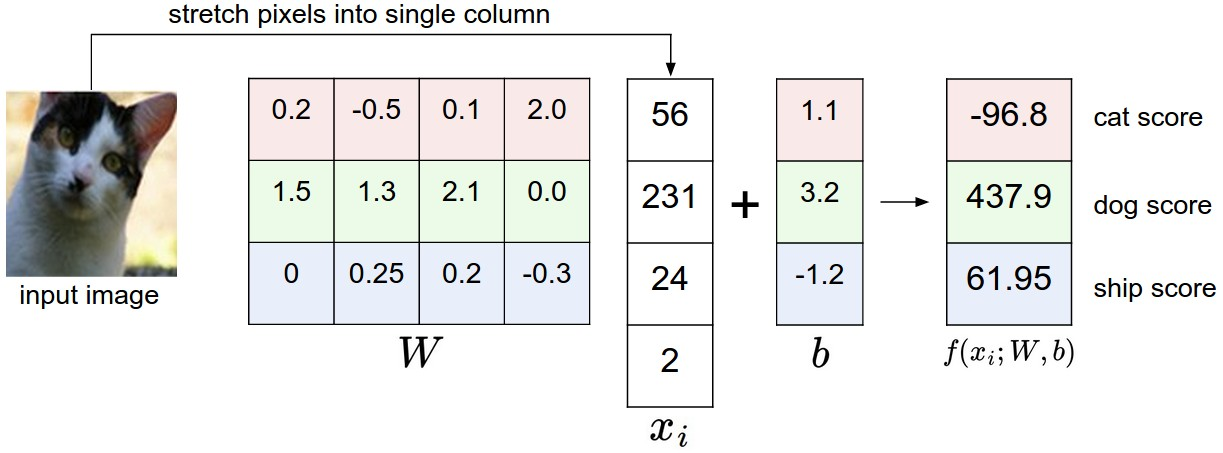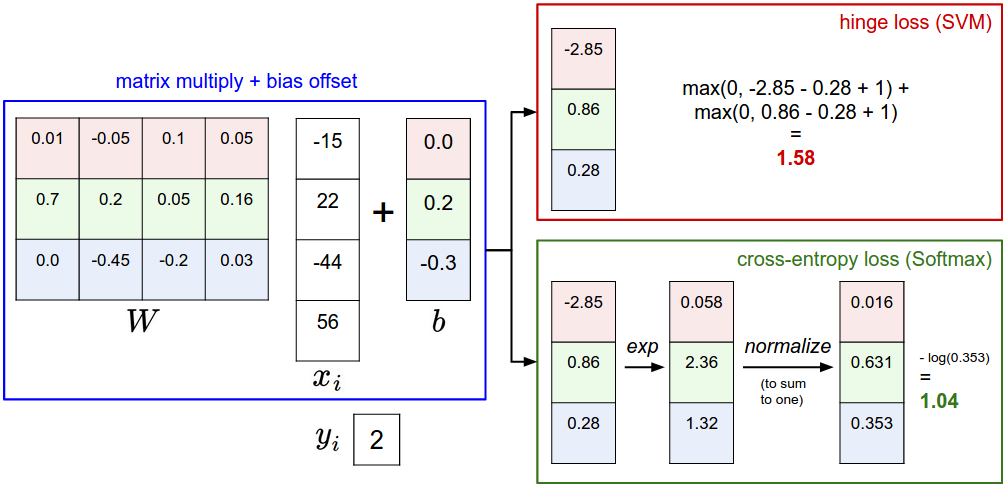# CS231n: Linear Classification

## 线性分类笔记

Posted by Jianfei on September 26, 2016

## CS231n Introduction

Stanford CS231n-Convolutional Neural Networks for Visual Recognition(Winter 2016)是一门讲述CNN在图像识别和分类中应用的课程，由Prof. Feifei Li授课。它包括两个模块，分别是Neural Networks和Convolutional Neural Networks，课程可用资源为Course Notes知乎翻译版。另外在Youtube中有完整版的Lecture Video。使用的数据集来自多伦多大学的Cifar-10

### 评分函数

$f(x_i,W,b)=Wx_i+b$首先将图像像素拉伸为一个列向量，与W进行矩阵乘，然后得到各个分类的分值。这个过程实际上是在高维空间当中的线性二值分类。线性分类器中的权重矩阵每一行对应着一个分类的模板，而图像不同分类的得分是通过计算图像与模板的内积得到的。模板是通过训练集训练得到的，而内积衡量了距离（相似度）。另外，为了便于计算，我们把偏差向量放到权重矩阵的最后一列，并在测试图像的列向量中增加一个单位末尾元素，### 损失函数

SVM损失是让正确分类始终比错误分类的得分高出一个阈值$\Delta$。给出第$i$个数据包括$x_i, y_i$，评分函数得到第$j$个类别的评分$s_j=f(x_i,W)_j$。那么这个数据的损失函数就是：

$L_i=\sum_{j\neq y_i}max(0,s_j-s_{y_i}+\Delta)$

$L_i=\sum_{j\neq y_i}max(0,w_j^T x_i-w_{y_i}^T x_i+\Delta)$

$R(W)=\sum_k \sum_l W_{k,l}^2$

$L=\frac{1}{N}\sum_i L_i + \lambda R(W)$

• 最大边界通常被设置成$\Delta=1.0$，因为它与另一个超参数$\lambda$同时控制了数据损失与正则化损失的权衡比例。这就决定了实际边界的大小。所以我们只需要确定一个来训练另一个正则化强度参数就可以了。

• 这里的SVM是多种SVM公式中的一种，还有常见的是One-Vs-All(OVA) SVM，它对每个类与其他类训练独立的二元分类器。还有一种更少见的All-Vs-All(AVA) SVM。有一种有趣的SVM称为Structured SVM，它将正确分类的分类分值和非正确分类中的最高分值的边界最大化。

### Softmax分类器

Softmax分类器可以理解为逻辑回归分类对多类问题的一般化归纳。保持评分函数不变，将评分视为每个分类未归一化的对数概率。折叶损失换为交叉熵损失(cross-entropy loss)，数据集的损失依然表示为损失值和正则化损失之和。交叉熵损失公式如下：

$L_i=-log(\frac{e^{f_{y_i}}}{\sum_j e^{f_j}})$

• 信息理论：在信息论中，真实分布$p$与估计分布$q$之间的交叉熵定义为：
$H(p,q)=-\sum_x p(x) logq(x)$

$H(p,q)=H(p)+D_{K,L}(p||q)$

• 概率论：评分函数我们看作是没有归一化的对数概率：
$P(y_i|x_i,W)=\frac{e^{f_{y_i}}}{\sum_j e^{f_j}}$

softmax函数将其转化为了归一化的概率分布，最小化正确分类的负对数概率相当于最大似然估计（MIE），而正则化部分$R(W)$可以被看作权重矩阵W的高斯先验，那么这里做的就是最大后验估计（MAP）。

• 实践技巧：在实际编程时，会遇到指数和过大的问题，所以要将数值进行归一化，也就是在分式的分子分母都乘以一个常熟C，使得数值计算更加稳定。通常我们取$logC=-max_j f_j$，将向量$f$中的数值进行平移使得最大值为0。
$\frac{Ce^{f_{y_i}}}{C\sum_j e^{f_j}}=\frac{e^{f_{y_i}}+logC}{\sum_j e^{f_j}+logC}$

### 对比SVM与Softmax

SVM给出是否是该分类的判断，而softmax给出了对于不同分类准确率的把握。而可能性分布的集中与离散程度由正则化参数$\lambda$决定。当正则化参数越大，那么权重W会被惩罚的越多，那么它的权重数值就会更小，而输出的概率分布就会更加均匀离散。